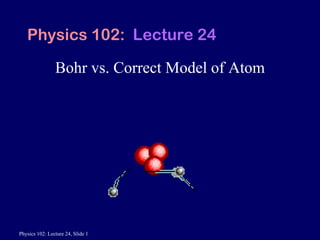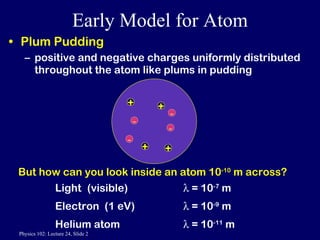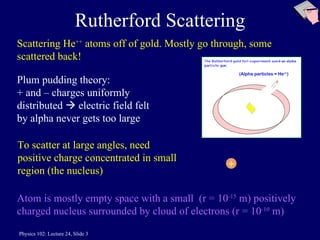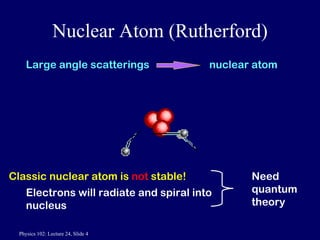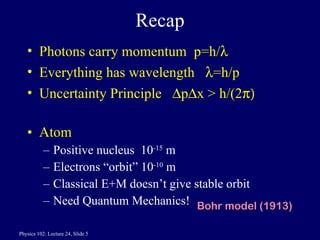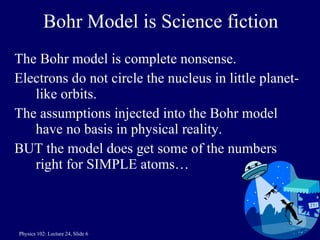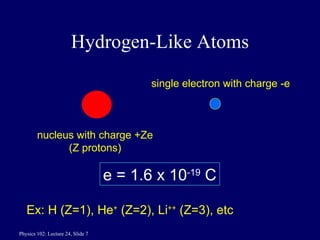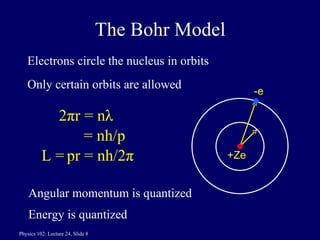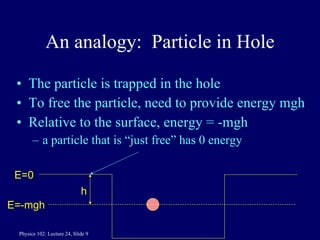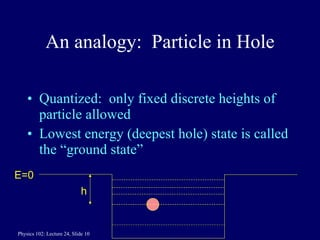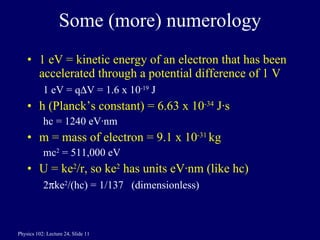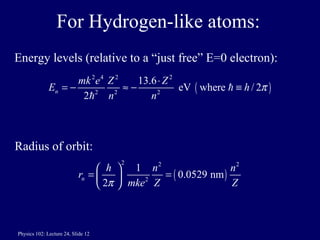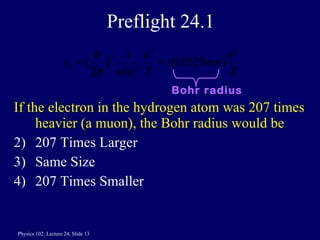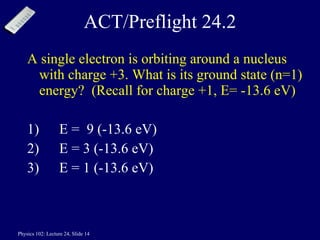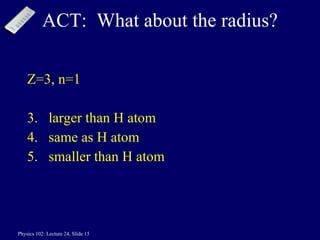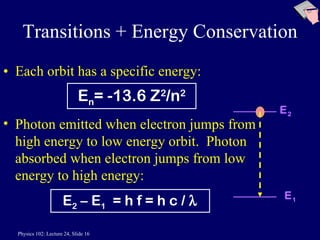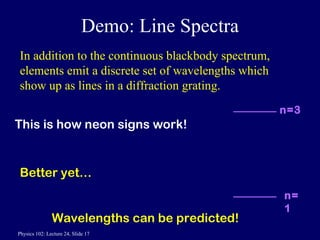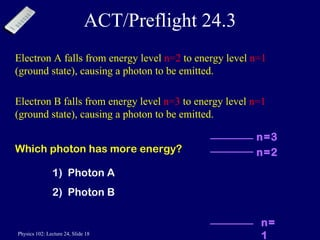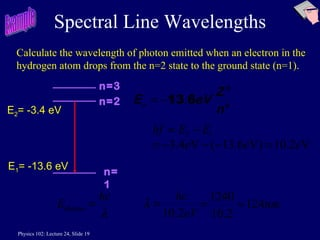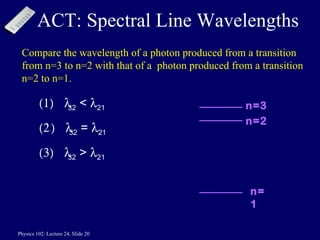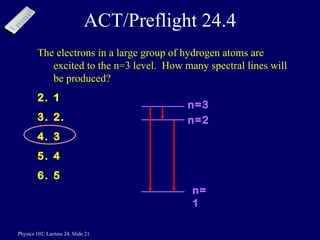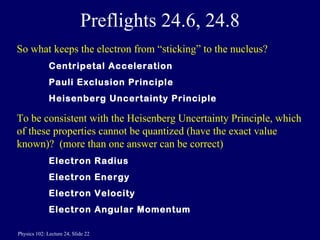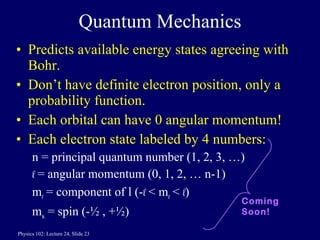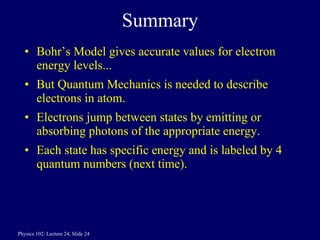1 de 24

### Lect24 handout

1. Bohr vs. Correct Model of Atom Physics 102: Lecture 24
2. Rutherford Scattering Scattering He ++ atoms off of gold. Mostly go through, some scattered back! Atom is mostly empty space with a small (r = 10 -15 m) positively charged nucleus surrounded by cloud of electrons (r = 10 -10 m) Plum pudding theory: + and – charges uniformly distributed  electric field felt by alpha never gets too large To scatter at large angles, need positive charge concentrated in small region (the nucleus) + (Alpha particles = He ++ )
3. Nuclear Atom (Rutherford) Classic nuclear atom is not stable! Electrons will radiate and spiral into nucleus Need quantum theory Large angle scatterings nuclear atom
4. Hydrogen-Like Atoms nucleus with charge +Ze (Z protons) single electron with charge -e e = 1.6 x 10 -19 C Ex: H (Z=1), He + (Z=2), Li ++ (Z=3), etc
5. The Bohr Model Electrons circle the nucleus in orbits Only certain orbits are allowed 2 π r = n λ = nh/p pr = nh/2 π Angular momentum is quantized -e +Ze L = Energy is quantized
6. Demo: Line Spectra In addition to the continuous blackbody spectrum, elements emit a discrete set of wavelengths which show up as lines in a diffraction grating. Better yet… Wavelengths can be predicted! This is how neon signs work! n=3 n=1
7. ACT/Preflight 24.3 Electron A falls from energy level n=2 to energy level n=1 (ground state), causing a photon to be emitted. Electron B falls from energy level n=3 to energy level n=1 (ground state), causing a photon to be emitted. Which photon has more energy? 1) Photon A 2) Photon B n=2 n=3 n=1
8. Spectral Line Wavelengths Calculate the wavelength of photon emitted when an electron in the hydrogen atom drops from the n=2 state to the ground state (n=1). E 1 = -13.6 eV E 2 = -3.4 eV Example n=2 n=3 n=1
9. ACT: Spectral Line Wavelengths Compare the wavelength of a photon produced from a transition from n=3 to n=2 with that of a photon produced from a transition n=2 to n=1.  32 <  21  32 =  21  32 >  21 n=2 n=3 n=1
10. Preflights 24.6, 24.8 So what keeps the electron from “sticking” to the nucleus? Centripetal Acceleration Pauli Exclusion Principle Heisenberg Uncertainty Principle To be consistent with the Heisenberg Uncertainty Principle, which of these properties cannot be quantized (have the exact value known)? (more than one answer can be correct) Electron Radius Electron Energy Electron Velocity Electron Angular Momentum

### Notas del editor

1. 1
2. Fundamental assumption of Bohr model: atomic levels are quantized
3. ).
4. Mention: Quantized energy means particle cannot be anywhere in the hole but only at discrete heights (or energy levels).
5. check numerology: 511000/(2*137*137)=13.6 eV 1240 *137/(2*pi*511000)=0.0529 nm
6. Again, basically guessed on this one. Might do it as an ACT
7. greater attraction, smaller radius, more binding This is doubly ionized Li